Python | Pandas dataframe.reindex_axis()

• Last Updated : 22 Nov, 2018

Python is a great language for doing data analysis, primarily because of the fantastic ecosystem of data-centric python packages. Pandas is one of those packages and makes importing and analyzing data much easier.

Pandas dataframe.reindex_axis() function Conform input object to new index. The function populates NaN values in locations having no value in the previous index. It also provides a way to fill the missing values in the dataframe. A new object is produced unless the new index is equivalent to the current one and copy=False

Syntax:
Syntax: DataFrame.reindex_axis(labels, axis=0, method=None, level=None, copy=True, limit=None, fill_value=nan)

Parameters :
labels : New labels / index to conform to. Preferably an Index object to avoid duplicating data
axis : {0 or ‘index’, 1 or ‘columns’}
method : {None, ‘backfill’/’bfill’, ‘pad’/’ffill’, ‘nearest’}, optional
copy : Return a new object, even if the passed indexes are the same
level : Broadcast across a level, matching Index values on the passed MultiIndex level
limit : Maximum number of consecutive elements to forward or backward fill
tolerance : Maximum distance between original and new labels for inexact matches. The values of the index at the matching locations most satisfy the equation abs(index[indexer] – target) <= tolerance.

Returns : reindexed : DataFrame

Example #1: Use reindex_axis() function to reindex the dataframe over the index axis. By default values in the new index that do not have corresponding records in the dataframe are assigned NaN.
Note : We can fill in the missing values using ‘ffill’ method

 # importing pandas as pdimport pandas as pd  # Creating the dataframe df = pd.DataFrame({"A":[1, 5, 3, 4, 2],                    "B":[3, 2, 4, 3, 4],                   "C":[2, 2, 7, 3, 4],                   "D":[4, 3, 6, 12, 7]},                   index =["A1", "A2", "A3", "A4", "A5"])  # Print the dataframedf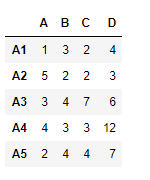Let’s use the dataframe.reindex_axis() function to reindex the dataframe over the index axis

 # reindexing with new index valuesdf.reindex_axis(["A1", "A2", "A4", "A7", "A8"], axis = 0)

Output :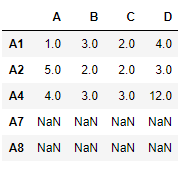Notice the output, new indexes are populated with NaN values, we can fill in the missing values using ‘ffill’ method.

 # filling the missing values using ffill methoddf.reindex_axis(["A1", "A2", "A4", "A7", "A8"],                      axis = 0, method ='ffill')

Output :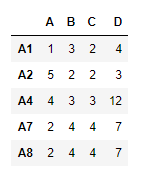Notice in the output, the new indexes has been populated using the “A5” row.

Example #2: Use reindex_axis() function to reindex the column axis

 # importing pandas as pdimport pandas as pd  # Creating the dataframe df = pd.DataFrame({"A":[1, 5, 3, 4, 2],                   "B":[3, 2, 4, 3, 4],                   "C":[2, 2, 7, 3, 4],                   "D":[4, 3, 6, 12, 7]},                    index =["A1", "A2", "A3", "A4", "A5"])  # reindexing the column axis with# old and new index valuesdf.reindex_axis(["A", "B", "D", "E"], axis = 1)

Output :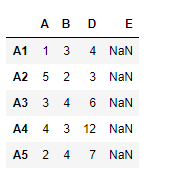Notice, we have NaN values in the new columns after reindexing, we can take care of the missing values at the time of reindexing. By using ffill method we can forward fill the missing values.

 # reindex the columns# we fill the missing values using ffill methoddf.reindex_axis(["A", "B", "D", "E"], axis = 1, method ='ffill')

Output :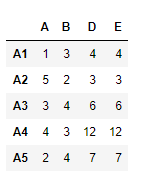My Personal Notes arrow_drop_up